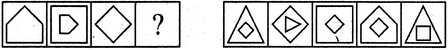# Non Verbal Reasoning - Analogy - Discussion

### Discussion :: Analogy - Section 2 (Q.No.1)

Each of the following questions consists of two sets of figures. Figures A, B, C and D constitute the Problem Set while figures 1, 2, 3, 4 and 5 constitute the Answer Set. There is a definite relationship between figures A and B. Establish a similar relationship between figures C and D by selecting a suitable figure from the Answer Set that would replace the question mark (?) in fig. (D).

1.

Select a suitable figure from the Answer Figures that would replace the question mark (?).(A)     (B)      (C)     (D)                  (1)      (2)      (3)      (4)      (5)

 [A]. 1 [B]. 2 [C]. 3 [D]. 4 [E]. 5

Explanation:

The figure rotates 90oCW; gets reduced in size and also gets enclosed in a figure with one less number of sides.

 Swati said: (Nov 3, 2014) The figure is not decreasing by one side only the size decreases.

 Asc said: (Jul 23, 2015) The figure rotates CW surely.

 Nemo said: (Sep 28, 2015) Answer should be 5 as per the explanation given. The rotation of the figure happens before enclosing inside a figure with a side lesser.

 Sumit said: (Mar 10, 2017) I think the answer should be 5.

 Amrit said: (May 28, 2017) Answer should have been 5.

 Shashank said: (Sep 6, 2017) Answer is 5.

 Vatsal said: (Apr 29, 2018) The correct answer is 5.

 Pavan said: (Jun 23, 2019) The answer is 5.

 G Yogananda Naidu said: (May 22, 2020) The answer is 5.

 Rohan Tyagi said: (Nov 3, 2020) The answer should be 5.

 Shakil Sagar said: (Nov 11, 2020) The answer should be 5 because figure rotates 90 CW and become sequence.

 Vicky2565 said: (Aug 2, 2021) The answer is A because on E the rhombus only ROTATES 45*CW. On D, the shape surrounding the rhombus has too many sides. On B, it's obvious the there is a different shape in the middle. On C, you can't have an equal amount of sides in each shape on this figure. This leaves A, ROTATING 90*CW, having one less amount of sides on the shape surrounding, and the shape on the third figure shrinking. Hope this helps settle that the answer is not E.